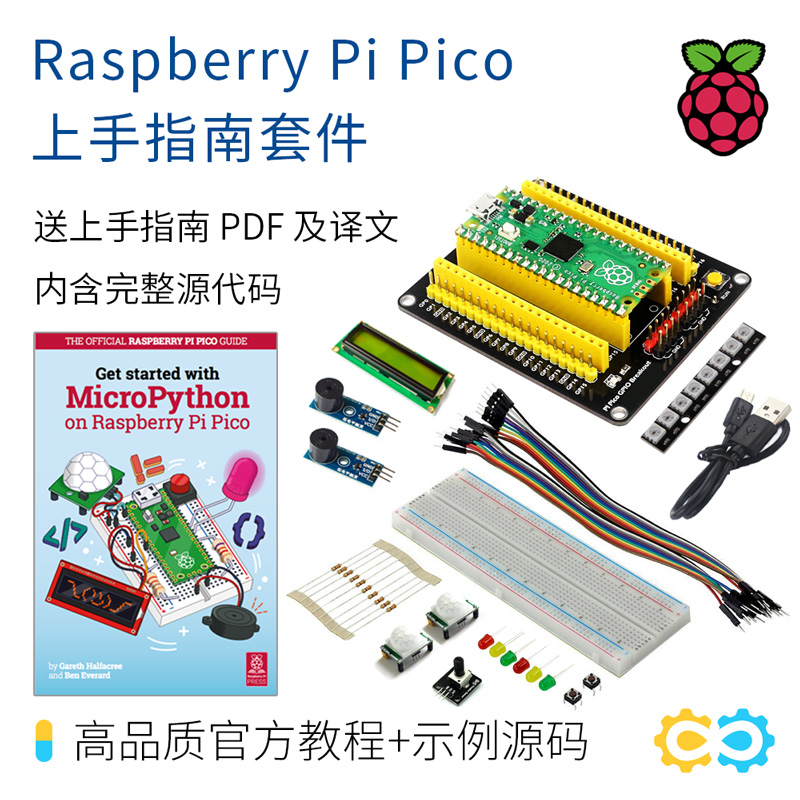# 使用树莓派 Pico 内置的温度传感器```import machine
import utime
```

```sensor_temp = machine.ADC(4)
```

```conversion_factor = 3.3 / (65535)
```

```while True:
```

```temperature = 27 - (reading - 0.706)/0.001721
```

```import machine
import utime

conversion_factor = 3.3 / (65535)

while True:
temperature = 27 - (reading - 0.706)/0.001721
print(temperature)
utime.sleep(2)
```#### 1 评论

1. 你这个没解决温度波动太大问题